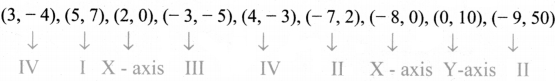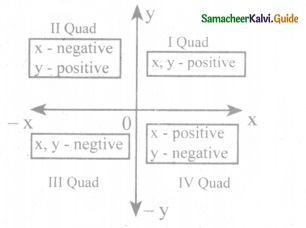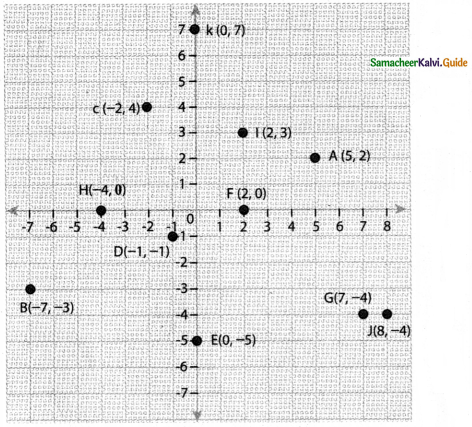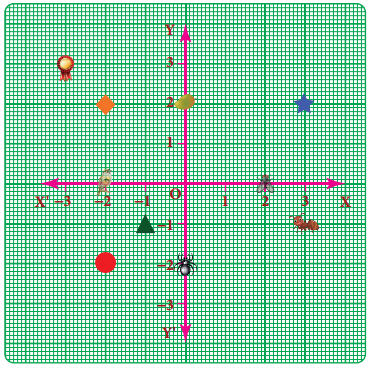Tamilnadu State Board New Syllabus Samacheer Kalvi 8th Maths Guide Pdf Chapter 3 Algebra Ex 3.8 Text Book Back Questions and Answers, Notes.

## Tamilnadu Samacheer Kalvi 8th Maths Solutions Chapter 3 Algebra Ex 3.8

Question 1.
Fill in the blanks:
(i) X- axis and Y-axis intersect at _________ .
Origin (0,0)

(ii) The coordinates of the point in third quadrant are always _________ .
negative(iii) (0, -5) point lies on _________ axis.
Y-axis

(iv) The x- coordinate is always ______ on the y-axis.
Zero

(v) ___________ coordinates are the same for a line parallel to Y-axis.
X

Question 2.
Say True or False:
(i) (-10,20) lies in the second quadrant.
True
Hint:
(-10, 20)
x = -10, y = 20
∴ (-10, 20) lies in second quadrant – True

(ii) (-9, 0) lies on the x-axis.
True
Hint:
(-9, 0) on x – axis. Y- coordinate is always zero.
∴ (-9, 0) lies on x axis – True(iii) The coordinates of the origin are (1,1).
False
Hint:
Coordinate of origin is (0, 0), not (1, 1). Hence – False

Question 3.
Find the quadrants without plotting the points on a graph sheet.
(3, -4), (5, 7), (2, 0), (-3, -5), (4, -3), (-7, 2), (-8, 0), (0, 10), (-9, 50).If X & y coordinate are positive – I quad
If x is positive,y is negative – IV quad
If x is negative, y is positive – II quad
If both are negative, then – III quadQuestion 4.
Plot the following points in a graph sheet.
A(5, 2), B(-7, -3), C(-2, 4), D(-1, -1), E(0, -5), F(2, 0), G(7, -4), H(-4, 0), I(2, 3), J(8, -4), K(0, 7).